International
Tables for
Crystallography
Volume C
Mathematical, physical and chemical tables
Edited by E. Prince

International Tables for Crystallography (2006). Vol. C, ch. 8.6, pp. 710-711

## Section 8.6.1. Basic theory

A. Albinatia and B. T. M. Willisb

aIstituto Chimica Farmaceutica, Università di Milano, Viale Abruzzi 42, Milano 20131, Italy, and bChemical Crystallography Laboratory, University of Oxford, 9 Parks Road, Oxford OX1 3PD, England

### 8.6.1. Basic theory

| top | pdf |

The model of the structure is refined by least-squares minimization of the residual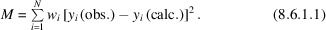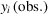is the intensity measured at a point i in the diffraction pattern corrected for the background intensity,is its weight, and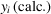is the calculated intensity. If the background at each point is assumed to be zero, and if the only source of error in measuring the intensities is that from counting statistics, the weight is given by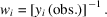The summation in (8.6.1.1)runs over all N data points. The number of data points can be arbitrarily increased by reducing the interval between adjacent steps. However, this does not necessarily imply an improvement in the standard uncertainties (s.u.'s; see Section 8.1.2) of the structural parameters (see Subsection 8.6.2.5), which are dependent on the number of linearly independent columns in the design matrix [equation (8.1.2.3)]. The number of independent observations in a powder pattern is determined by the extent of overlapping of adjacent reflections. An intuitive argument for estimating this number has been proposed by Altomare et al. (1995) and a more rigorous statistical estimate has been described by Sivia (2000). The strategy for choosing the number of steps and apportioning the available counting time has been discussed by McCusker et al. (1999) and references therein. The relation between counting statistics and the s.u.'s has been discussed by Baharie & Pawley (1983) and by Scott (1983).

The calculated intensity is evaluated using the equation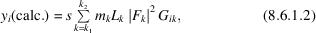where s is a scale factor,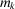is the multiplicity factor for the kth reflection,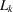is the Lorentz–polarization factor,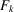is the structure factor and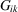is the peak-shape function' (PSF). The summation in (8.6.1.2)is over all nearby reflections,to, contributing to a given data point i.

A fundamental problem of the Rietveld method is the formulation of a suitable peak-shape function. For X-rays, a mixture of Gaussian and Lorentzian components is sometimes used (see Subsection 8.6.2.2). For neutrons, it is easier to find a suitable analytical function, and this is, perhaps, the main reason for the initial success of neutron Rietveld analysis. For a neutron diffractometer operating at a fixed wavelength and moderate resolution, the PSF is approximately a Gaussian of the form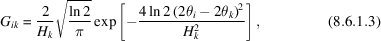where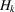is the full width at half-maximum (FWHM) of the peak,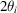is the scattering angle at the ith point, and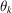is the Bragg angle for reflection k.

The angular dependence of the FWHM for a Gaussian peak-shape function can be written in the form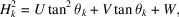where U, V and W are half-width parameters independent of(Caglioti, Paoletti & Ricci, 1958). To allow for intrinsic sample broadening and instrumental resolution, U, V and W are treated as adjustable variables in the least-squares refinement. The tails of a Gaussian peak decrease rapidly with distance from the maximum, and the intensity at one-and-a-half times the FWHM from the peak is only about 0.2% of the intensity at the peak. Thus, no large error is introduced by assuming that the peak extends over a range of approximately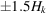and is cut off outside this range. The FWHM for the Lorentzian (see Subsection 8.6.2.2) can be modelled by the relation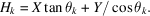The Lorentzian function extends over a much wider range than the Gaussian. A more flexible approach to this line-broadening problem is described by McCusker et al. (1999).

The least-squares parameters are of two types. The first contains the usual structural parameters: for example, fractional coordinates of each atom in the asymmetric unit and the corresponding isotropic or anisotropic displacement parameters. The second type represents profile parameters' which are not encountered in a least-squares refinement of single-crystal data. These include the half-width parameters and the dimensions of the unit cell. Further parameters may be added to both groups allowing for the modelling of the background and for the asymmetry of the reflections. The maximum number of parameters that can be safely included in a Rietveld refinement is largely determined by the quality of the diffraction pattern, but intrinsic line broadening will set an upper limit to this number (Hewat, 1986).

The following indicators are used to estimate the agreement with the model during the course of the refinement.

Profile R factor: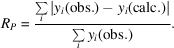Weighted profile R factor: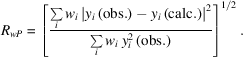Bragg R factor: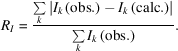Expected R factor: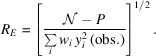Ik is the integrated intensity of the kth reflection,is the number of independent observations, and P is the number of refined parameters. The most important indicators are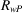and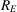. The ratio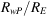is the so-called `goodness-of-fit', χ2: in a successful refinement χ2 should approach unity. The Bragg R factor is useful, since it depends on the fit of the structural parameters and not on the profile parameters.

### References

Altomare, A., Cascarano, G., Giacovazzo, C., Guagliardi, A., Moliterni, A. G., Burla, M. C. & Polidori, G. (1995). On the number of statistically independent observations in powder diffraction. J. Appl. Cryst. 15, 361–374.
Baharie, E. & Pawley, G. S. (1983). Counting statistics and powder diffraction scan refinements. J. Appl. Cryst. 16, 404–406.
Caglioti, G., Paoletti, A. & Ricci, F. P. (1958). Choice of collimators for a crystal spectrometer for neutron diffraction. Nucl. Instrum. Methods, 3, 223–228.
Hewat, A. W. (1986). High-resolution neutron and synchrotron powder diffraction. Chem. Scr. 26A, 119–130.
McCusker, L. B., Von Dreele, R. B., Cox, D. E., Louer, D. & Scardi, P. (1999). Rietveld refinement guidelines. J. Appl. Cryst. 32, 36–50.
Scott, H. G. (1983). The estimation of standard deviations in powder diffraction refinement. J. Appl. Cryst. 16, 589–610.
Sivia, D. S. (2000). The number of good reflections in a powder pattern. J. Appl. Cryst. 33, 1295–1301.